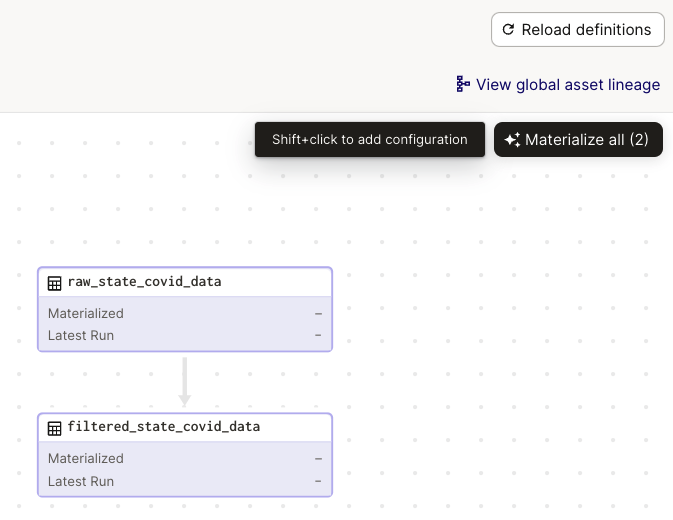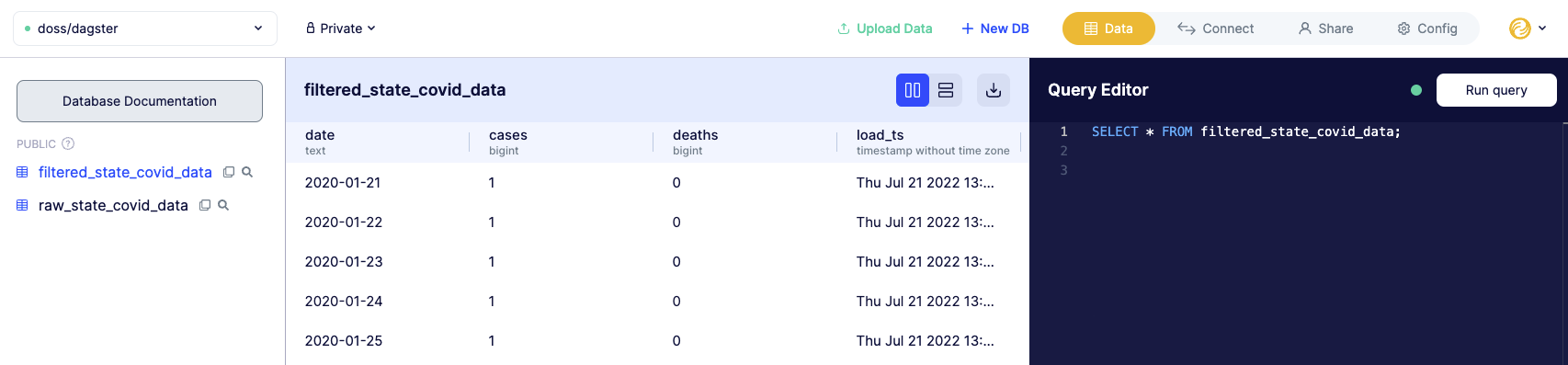# Dagster

Dagster is an open-source library for building systems like ETL processes and ML pipelines, which Dagster calls "data applications". Dagster data applications are defined as "a graph of functional computations that produce and consume data assets".

bit.io databases can be used to provide zero-config storage of assets within these data applications, enabling developers and analysts to build and query directly against the assets. In case this sounds a bit abstract, we'll walk through a simple example showing an ETL process using The New York Time's open-source COVID data.

# A minimal Dagster graph

Before connecting, we need to define an example Dagster graph. Our example graph contains two assets:

1. `raw_state_covid_data` - A table of the raw data from The NYT's GitHub.
2. `filtered_state_covid_data` - A filtered table containing select columns for only Washington State.

Each asset is defined as a Python operation function with an `@asset` decorator. Dependencies between assets are defined implicitly using the parameters defined for each "op" function — for example, the object returned by `raw_state_covid_data` is defined as a parameter for `filtered_state_covid`. You can read more about assets and op functions in the Dagster docs.

``````import io
import pandas as pd
import requests

from dagster import asset

@asset
def raw_state_covid_data():
# we directly download the raw dataset in this op, so this asset
# has no internal dependencies
url = "https://raw.githubusercontent.com/nytimes/covid-19-data/master/us-states.csv"
r = requests.get(url, timeout=5)
data = r.content.decode('utf-8')
df =  pd.read_csv(io.StringIO(data), low_memory=False)
return df

@asset
def filtered_state_covid_data(raw_state_covid_data):
# here, we filter both on column and row
keep_cols = ['date', 'cases', 'deaths']
wa_state_filter = raw_state_covid_data['state'] == 'Washington'
return raw_state_covid_data.loc[wa_state_filter, keep_cols]
``````

We can also visualize the defined graph using the Dagit web UI.

# Connecting to bit.io with Dagster

Using bit.io, we can persist each asset in our graph as a queryable, sharable database table on bit.io. In order to connect to Dagster with bit.io, you'll need to find your PosgreSQL connection credentials from the bit.io Connect Tab of the database to which you would like to connect. You can find everything you need to know about your PostgreSQL credentials here.

Using your bit.io connection string, you can configure a custom Dagster `IOManager`, `BitIOManager` that reads and writes assets to bit.io database tables. A Dagster `IOManager` requires two methods:

1. `load_input` is used to load an asset from storage as a Python object using its key (which defaults to the op function name in `assets.py`.
2. `handle_output` is used to write the Python object for an asset to storage.

`BitIOManager` uses pandas DataFrames for the Python objects and bit.io tables for storage. In this example, the raw data is downloaded from GitHub as a csv file, read into a DataFrame, and then loaded to a bit.io table named `raw_state_covid_data` in bit.io. Then, `raw_state_covid_data` is downloaded again, filtered, and written to a second bit.io table named `filtered_state_covid_data`.

The `BitIOManager` also automatically adds a `load_ts` (timestamp) column when loading to bit.io so that consumers know when the data was last updated. We also define a custom insert method for faster uploads to bit.io, which you can read more about in our pandas docs.

``````import csv
import datetime
from io import StringIO
import os

import pandas as pd
from sqlalchemy import create_engine
from dagster import (
IOManager,
IOManagerDefinition,
)

engine = create_engine(PG_STRING, pool_pre_ping=True)

class BitDotIOManager(IOManager):
schema = "public"

def _get_table_name(self, asset_key) -> str:
return "".join(asset_key.path)

def handle_output(self, context, df):
table_name = self._get_table_name(context.asset_key)
with engine.begin() as conn:
df.to_sql(
table_name,
conn,
schema=self.schema,
index=False,
if_exists="replace",
method=psql_insert_copy,
)

table_name = self._get_table_name(context.asset_key)
with engine.begin() as conn:
table_name,
conn,
schema=self.schema,

# Custom insert method for DataFrame.to_sql
def psql_insert_copy(table, conn, keys, data_iter):
# gets a DBAPI connection that can provide a cursor
dbapi_conn = conn.connection
with dbapi_conn.cursor() as cur:
s_buf = StringIO()
writer = csv.writer(s_buf)
writer.writerows(data_iter)
s_buf.seek(0)

columns = ', '.join(f'"{k}"' for k in keys)
table_name = f'"{table.schema}"."{table.name}"'
sql = f'COPY {table_name} ({columns}) FROM STDIN WITH CSV'
cur.copy_expert(sql=sql, file=s_buf)
``````

Finally, we can register our custom `BitIOManager` for use with the assets in our `assets.py` module as follows:

``````from dagster import (
materialize,
with_resources,
)

import assets

assets_with_io_manager = with_resources(
resource_defs={
"io_manager": IOManagerDefinition.hardcoded_io_manager(DataframeTableIOManager())
},
)

if __name__ == "__main__":
``````

The last few lines allow us to run `pipeline.py` as a Python script. We can also run the pipeline from the Dagit web UI by clicking the "Materialize all" button in the upper right.Finally, we can check our bit.io database and confirm that assets mirroring our pandas DataFrames have been stored as queryable, timestamped, bit.io database tables:# Using Dagster with bit.io

Using this connection example and the `BitIOManager` class, you can compose arbitrarily complex ETL and ML data pipelines while storing your tabular data assets as queryable, sharable tables in bit.io. This guide only scratches the surface of what you can do with bit.io and Dagster, so be sure to check out the bit.io and Dagster docs for additional features.

A copy of the full `pipeline.py` module is provided below:

``````import csv
import datetime
from io import StringIO

import pandas as pd
from sqlalchemy import create_engine
from dagster import (
materialize,
IOManager,
IOManagerDefinition,
AssetKey,
with_resources,
)

import assets

engine = create_engine(PG_STRING, pool_pre_ping=True)

class BitDotIOManager(IOManager):
# def _get_fs_path(self, asset_key: AssetKey) -> str:
#     rpath = os.path.join(*asset_key.path) + ".csv"
#     return os.path.abspath(rpath)
schema = "public"

def _get_table_name(self, asset_key: AssetKey) -> str:
return "".join(asset_key.path)

def handle_output(self, context, df):
# name is the name given to the Out that we're storing for
# fpath = self._get_fs_path(context.asset_key)
# df.to_csv(fpath)
table_name = self._get_table_name(context.asset_key)
with engine.begin() as conn:
df.to_sql(
table_name,
conn,
schema=self.schema,
index=False,
if_exists="replace",
method=psql_insert_copy,
)

# upstream_output.name is the name given to the Out that we're loading for
# fpath = self._get_fs_path(context.asset_key)
table_name = self._get_table_name(context.asset_key)
with engine.begin() as conn:
table_name,
conn,
schema=self.schema,

# Custom insert method for DataFrame.to_sql
def psql_insert_copy(table, conn, keys, data_iter):
# gets a DBAPI connection that can provide a cursor
dbapi_conn = conn.connection
with dbapi_conn.cursor() as cur:
s_buf = StringIO()
writer = csv.writer(s_buf)
writer.writerows(data_iter)
s_buf.seek(0)

columns = ', '.join(f'"{k}"' for k in keys)
table_name = f'"{table.schema}"."{table.name}"'
sql = f'COPY {table_name} ({columns}) FROM STDIN WITH CSV'
cur.copy_expert(sql=sql, file=s_buf)

assets_with_io_manager = with_resources(